## Project Outsourcing to a Freelancer CFD Simulation Engineer Team

MR-CFD, the freelancing CFD simulation Company, is ready to fulfill every Computational Fluid Dynamics project. Our services include industrial and academic purposes considering a wide range of CFD simulations by the ANSYS Fluent software, which serves three main categories of ConsultationTraining, and CFD SimulationOrder your project to be simulated in the shortest time and with the highest quality. The following flowchart represents the project order procedure:

## What is Radiative Heat Transfer?

Radiant heat, also known as thermal radiation, is the transfer of electromagnetic radiation, which describes photons’ heat exchange of energy. Radiant heat is a mechanism for heat transfer that does not require a medium in which it propagates (unlike convection and conduction). All substances above absolute zero have thermal energy, meaning that the particles in them have some form of motion. This motion of the particles contributes to the object’s temperature, with objects of “ordinary” temperatures (less than 1000 Kelvin) emitting their radiant heat primarily in the infrared spectrum of light. The photons emitted by these moving charged particles will travel at the speed of light until they hit another particle, which absorbs its energy as kinetic energy. Interacting systems at different temperatures will do so by exchanging radiant heat until they reach thermal equilibrium with one another.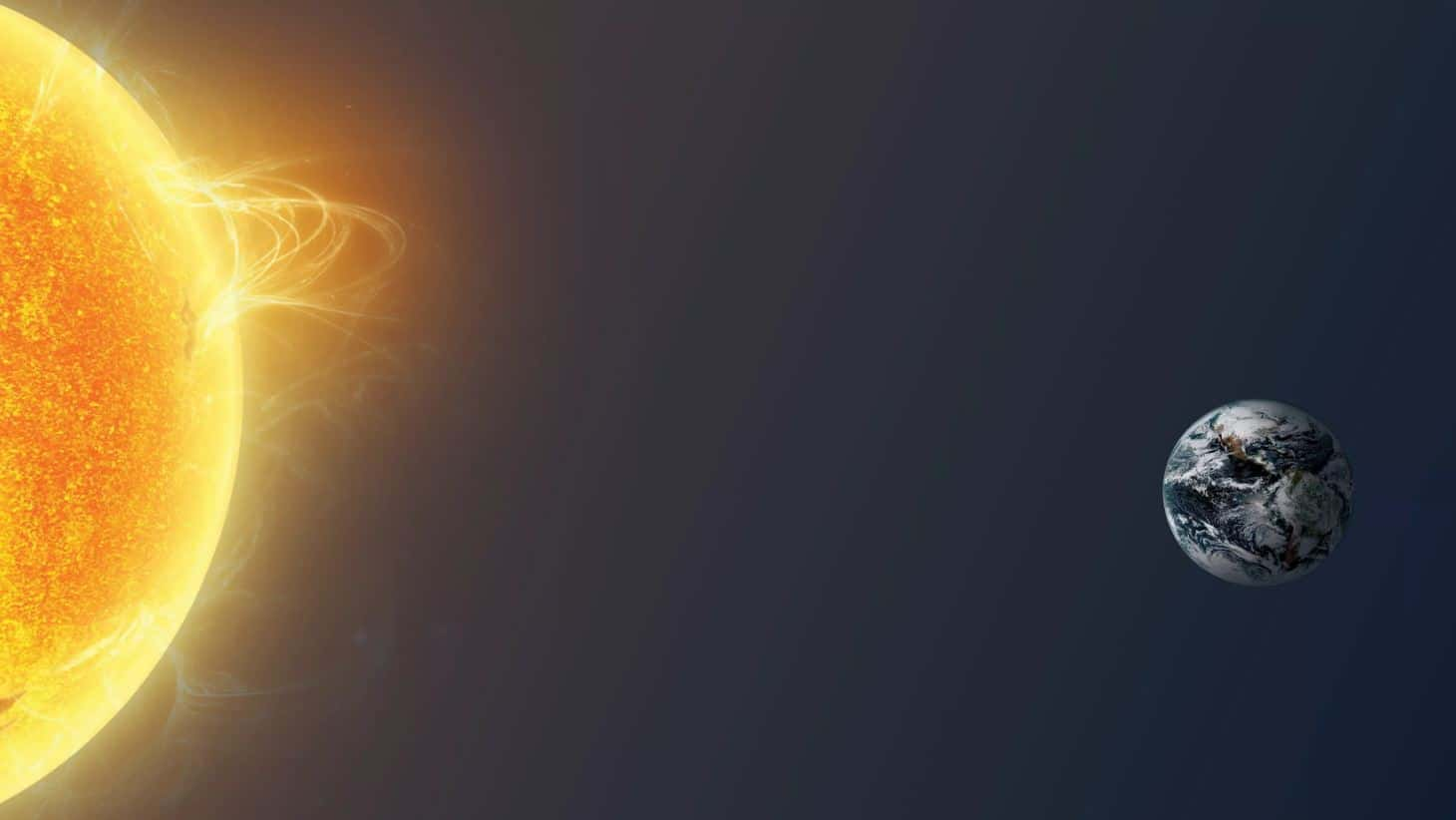## Radiative Heat Transfer Simulations in ANSYS Fluent Software

The Stefan-Boltzmann radiation law can explain the theory behind the heat transfer rate by emitted radiation.

Where σ = 5.67 × 10−8 J/s · m2 · K4 is the Stefan-Boltzmann constant, A is the surface area of the object, T2 and T1 are the absolute temperatures of the first and second systems in kelvin. The symbol ε stands for the object’s emissivity, which measures how well it radiates. An ideal jet-black (or black body) radiator has ε = 1, whereas a perfect reflector has ε = 0. Real objects fall between these two values. For example, tungsten light bulb filaments have an ε of about 0.5, and carbon black (a material used in printer toner) which have the (greatest known) emissivity of about 0.99.

## Radiative Heat Transfer Simulation in ANSYS Fluent

The radiation energy model is generally divided into two categories of radiation between different surfaces (including P1, S2S, DTRM, DO, and MC methods) and Solar-Ray Tracing. The Solar Calculator section defines the geographic region’s Latitude and Longitude, Time Zones, Hours, and Days of radiation. In the Sun Direction Factor section, the direction of the solar radiation can be determined. Direct Solar Irradiation is also considered (W.m-2). In addition, by enabling the Use Direction Computed from The Solar Calculator option, the direction of solar radiation to the model would be defined based on the input data to the Solar Calculator.

Other radiation heat transfer models are also available in ANSYS Fluent software, including:

• DO
• DRTM
• P1
• S2S
• Roseland
• MC
• solar ray tracing

### Discrete Ordinates Model (DO)

The discrete ordinates model (DO) is used for cases where the radiative heat transfer equations are solved for a discrete number of solid finite angles. Its advantages are that it is the most comprehensive radiation model, the solution accuracy is enhanced by better discretization, a stable method that results in a thermal energy balance for coarse discretization, and use for light scattering, semi-transparent environments, glossy sheets such as mirrors, and wavelength-dependent transmission using the gray environment while resolving it with a wide variety of angles is heavy for CPU, as a limitation of this model.

### Discrete Transfer Radiation Model (DTRM)

The discrete transfer radiation model (DTRM) is used for cases where the radiation emitted from a surface element in continuous and specified intervals of solid angles can be considered as a single ray. Its advantages are that the model is relatively simple, capable of increasing accuracy with the increasing number of rays, and can be applied to a wide range of optical thicknesses, while limitations are that not all pages are scattered, the effects of radiation scattering are not taken into account, and the solution is very heavy for the CPU.

### P1

The P1 model is used for cases where the independence of direction is integrated into the radiative heat transfer equations and thus results in a diffusion equation for random radiations. Its benefits include that the radiation heat transfer equation can be easily solved with a low CPU, including the effects of light scattering, such as the effects of particles or droplets of water or soot, and work well in applications such as combustion that have high optical thicknesses; while the limitations are that all plates are scattered, they may have low accuracy in some cases, especially in low-thickness geometries, and it is prone to predicting fluxes from local heat sources or sinks.

### Surface to Surface Radiation Model (S2S)

The Surface to Surface Radiation Model (S2S) is used for cases with no material environment or interference with radiation modeling in radiation-related situations. This radiation modeling includes solar collector systems, spacecraft back-heating systems, radiant space heaters, and automotive underground cooling systems. This model is also based on the view factor and is suitable for non-interference modes. Its limitations are that the required storage space increases rapidly when the number of pages is increased, assuming that the radiation is gray, all the pages are diffuse, and symmetric or intermittent boundary conditions are not used.

### Solar Ray Tracing

The solar ray-tracing model is called the ray tracing algorithm for thermal energy transfer from the sun, which is compatible with other radiation models and is used only for 3D models. Features of this model also include the solar directional vectors, the solar intensity (in terms of direct radiation and scattered emission), and the solar calculator to calculate the direction and intensity of direct radiation using maximum theoretical or equilibrium conditions.

In general, to select the best model of radiation heat transfer, the following is done:

• From a computational cost point of view:

P1 is reasonably accurate but low effort.

• From the point of view of solution accuracy:

DO and DTRM are more accurate.

• Optical Thickness point of view:

DTRM and DO are Suitable for Thin Optical Thickness (𝛂L “1), and P1 is Suitable for Thick Optical Thickness.

• In terms of dispersion or diffusion:

P1 and DO are suitable for diffusion.

• In terms of particle effects:

P1 and DO are suitable for the radiation exchange between gas and fine particles.

• From the viewpoint of local heat sources:

DO and DRTM are good enough with high radiation.

## Theta Divisions (NӨ) and Phi Divisions (Nφ)

Divisions (NӨ) and phi divisions (Nφ): The number of control angles used to break up every eighth of the annular space. There are only four one-eighth zones in two-dimensional models due to the symmetry lines in two directions. Thus a total of 4 * NӨNφ for the vector (with angles of Ө and φ) must be solved; As in 3D models, there are eight eighth-region, so a total of 8 * NӨNφ for the vector (with angles Ө and φ) must be solved. These angles are by default equal to two. However, more angles are suggested for more complex problems involving specific radiation exchange, which can usually be chosen from three to five. In general, increasing the number of these angles and better discretization will better resolve the effects of small geometrical features or strong spatial variables on temperature, increasing computational costs.

## Theta Pixel and Phi Pixel

Theta pixel and Phi pixel: Pixels have bumps on each surface. For gray matter radiation, 1 * 1 pixelation is sufficient; increasing the number of pixel insertions also increases computational cost but has less computational effort than angular segmentation.

## Non-gray Model

Generally, surfaces whose radiation is independent of the path are called diffuse surfaces, and surfaces whose radiation is independent of wavelength are called gray surfaces. Therefore, to simulate non-gray radiation models, it is necessary to define spectra of specific wavelengths (with start and end wavelengths in micrometers). Also, increasing the number of optical spectra increases the computational cost.

## Emissivity (Boundary Condition)

The proportion of energy emitted by a surface to the energy emitted by a black body (the body most fully absorbing and emitting, or in other words, no object at a given temperature and wavelength can be greater than the black body emits energy) at the same temperature, called the emissivity, which always has a value between zero and one. This diffusion coefficient is used for inlet and outlet boundaries. The rough surfaces also have random radiative reflections, and smooth surfaces such as mirrors have reflective boundary conditions. The semi-transparent boundary condition is used to model objects such as glass boards in the air, and the opaque model is used to model matte walls that behave like gray objects. Then we can determine the diffuse fraction, which is the fraction of the reflective radiation flux in the form of propagation. When it is equal to 1, all radiation is propagated. When it is equal to zero, that is poor reflection radiation.

### Roseland

The Roseland model is for situations with a very thick light environment. Unlike other radiation models, this model does not need to define the internal emissivity coefficient in the boundary condition section; the software considers this value equal to one by default. The advantages of this method include low computational cost and no need for transport equations. In contrast, its limitations include usability only in high light thickness environments and unusability in density-based solution models. Optical thickness is used as a criterion for expressing the amount of radiation intensity absorbed, equal to the absorption coefficient multiplied by the average value of the characteristic length of the object. So if the amount of light thickness was high (greater than three), the Roseland method is used, while the P-1 method for light thickness above one, the S2S method for zero light thickness, and the DO and DTRM are used for all values of light thicknesses. In terms of application, the Roseland method is more suitable for glass applications (due to its high absorption coefficient).

The solar load model activates the thermal load of solar energy. Solar ray tracing is an algorithm for transferring solar energy compatible with all the radiation models mentioned. Of course, this mode can only be used for 3D models. By selecting this model, the characteristics of solar radiation on surfaces and objects can be determined by defining longitude, latitude, and time zone in the global position section, defining the north and east directions of the object concerning the sun’s radiation in the section. Applied mesh orientation and definition of the days and hours of radiation in the date and time section. The direction of the sun’s rays can also be determined using the Cartesian coordinates in the sun direction vector. By selecting a solar calculator, we can define the values for direct sunlight irradiation and diffuse solar irradiation by the software itself based on our input data.

## Semi Transparent & Opaque Boundary Conditions

The semi-transparent boundary condition is used to model objects such as glass boards in the air, and the opaque model is used to model opaque walls that behave like gray objects. By activating the participation in the solar ray tracing option, the selected boundary condition is affected by the radiant forces of solar energy.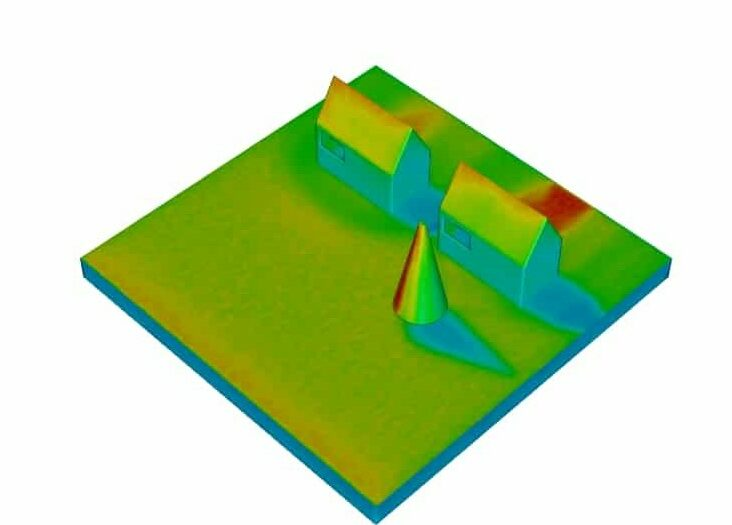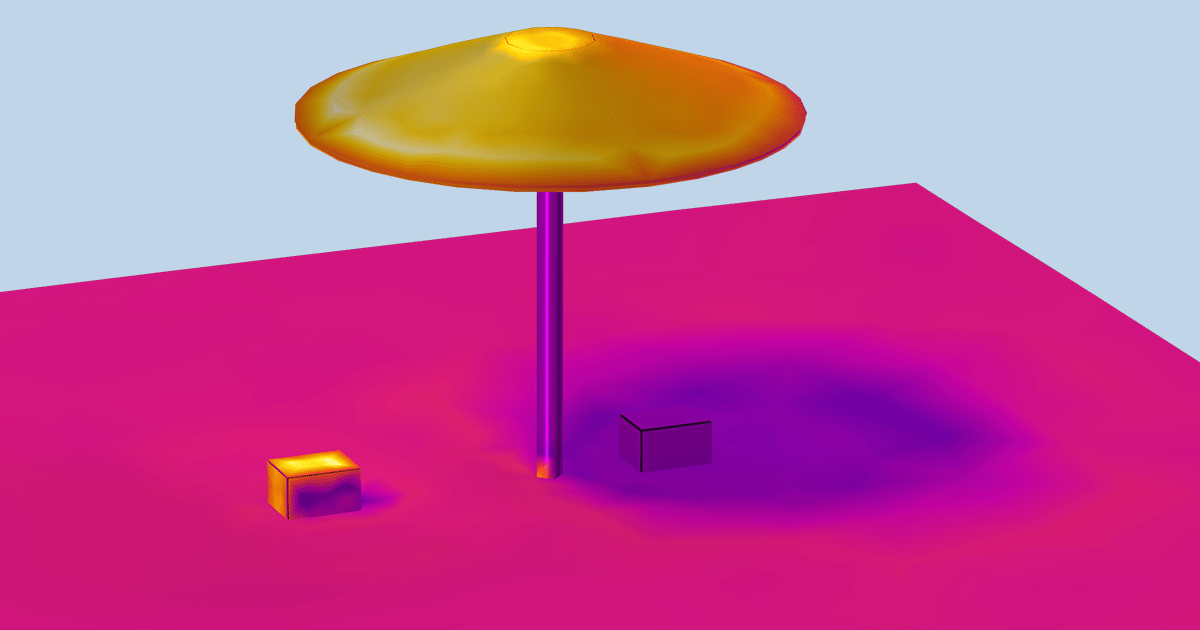To expand your knowledge about this model and its application in the industry and academic fields, and also how to use it inside the ANSYS Fluent software, you can enter the Radiation tab by clicking on the link to find more projects, for instance, using Rosseland method, the following simulation models a Dome-Shaped building which is under the effect of solar radiation.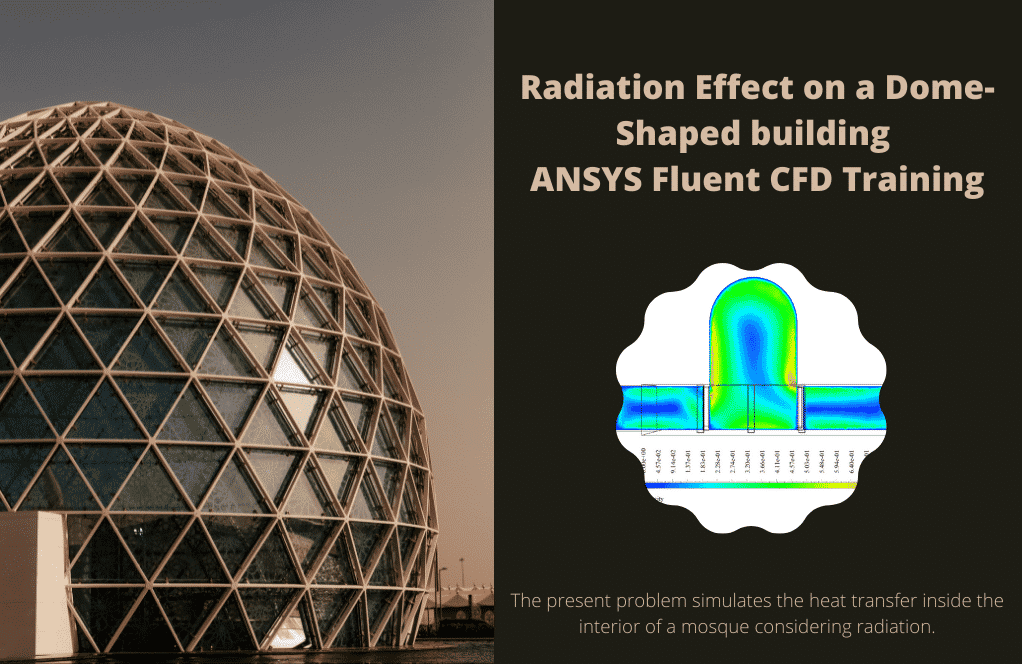Also, the following project concerns the radiation due to Biomass Combustion using the P1 radiation model.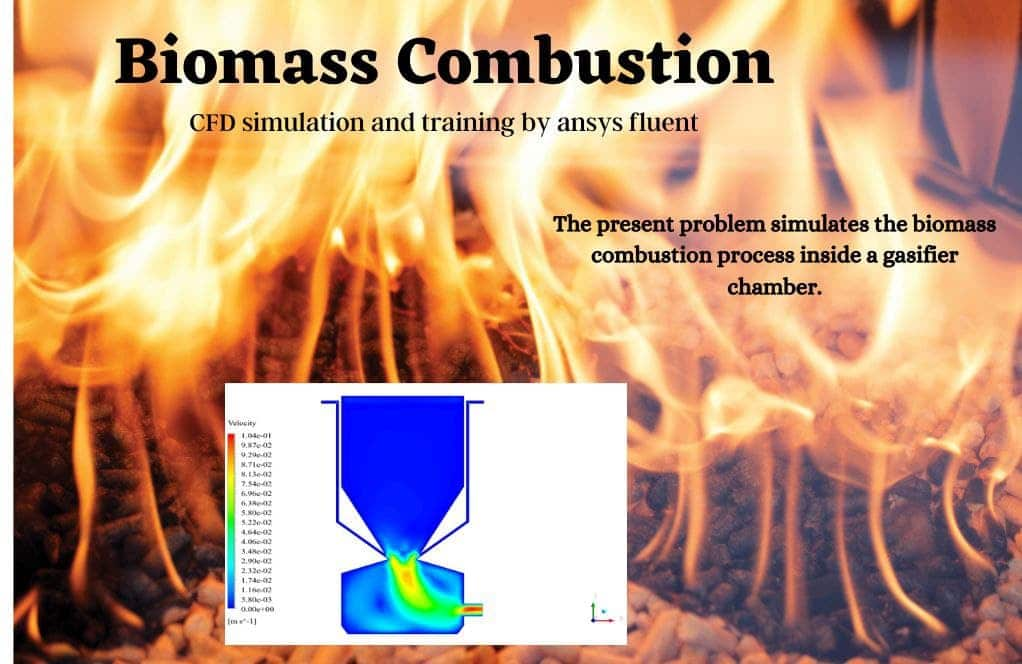https://www.mr-cfd.com/shop/gasifier-cfd-simulation-biomass-combustion/

## MR-CFD, an Expert in the Field of Heat Transfer Simulations

With several years of experience simulating various problems in various CFD fields using ANSYS Fluent software, the MR-CFD team is ready to offer extensive modeling, meshing, and simulation services. Simulation Services for radiative heat transfer simulations are categorized as follows:

• Solar Radiation at Different Hours
• Radiation Heat Transfer, Computer Room
• Radiation Heat Transfer in Combustion Chamber
• Solar Radiation Effect on a Gasoline Tank
• Finding outlet temperature in Solar collector in various flow rates and shape
• Using phase change material (PCM) for storing energy in a solar water heater

You may find the related products in the categories mentioned above in our CFD shop by clicking on the following link: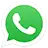Call On WhatsApp